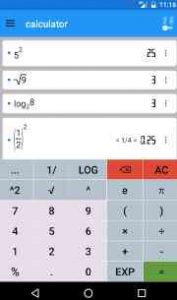# Mathematics – Powerful calculation software

## [App] MathematicsMathematics : formula calculation with variables, sums, products and sequences

solve equations (linear, quadratic, cubic, transpose)
plot functions, derivate, integrate, calculate roots and extremas (polynomial, rational, exponential, etc)
calculate tangent, asymptote, interception
reconstruction: calculate f(x) by given roots, points or extremas
f(x,y) plot in 3D
factoring (Bézout’s identity, Euler’s totient function, least common multiple, greatest common divisor)
modulo calculation with big integer and fractions
differential and integral calculus
curve sketching, limes, minima, maxima
linear algebra, vectors, matrices
unit conversion
number systems, binary/oct/decimal/hex calculator
complex number calculation
statistics (gaussian test, t test, binominal and normal distribution, standard deviation, average, sum, median)

Supported languages:
English, German, Francais, Espanol, Italian, Portuguese

Mathematics is a powerful calculation software for your android smartphone.

FEATURES

Calculate any formula you want and show them in a 2d or 3d plot. The natural display shows fractions, roots and exponents as you would expect it from mathematics.

In a few seconds you derivate or integrate your desired function, calculate the zero points of your function und show them in the function plot. See all maxima, minima or inflection points in one view.

The easy way of use allows you to solve linear equations in just a moment. Or transform your mathematical, physical or chemical equation to any unknown variable.

You often needs to calculate with binary, octal or hexadecimal number systems? No problem! You can mix them together in one calculation even by using decimal digits. But that’s not enough! You can also calculate with any other number system with base 2 to 18.

From time to time you may need to convert units to another one, like Celsius to Fahrenheit, miles to kilometre, inches to foot and so on.

You will also be able to calculate with vectors, matrices and determinants.

All this features are combined in this app and will make your mathematical life a lot easier.

Mathematics user reviews :

The app is great…. but due to some strange reasons I cant find integrals and differentials or maybe they are not included….I dont know

Really great. I can implement complex plots using this

Math explains why things add up. Excellent app.

This app is great. Besides doing simple calculations, it can also do complex calculations such as solving equations, plotting graphs, matrices and many other. It also shows expressions as real mathematical expressions. The only problem is that, the 3D graphs cannot be rotated and scaled. Please add that feature.

It is very helpful. Thank you very much. Keep up. I think saving plot along with function in same image will be more helpful.

So good!! practical, helpful, comprehensive, precise,… Definitely useful for engineering students. Thanks to developers for creating this app

Very useful and great helps a lot for understanding functions and other things !!

Best app for plotting, matrix multiplication and many more to explore. I just love this app!!

Very great math app for elementary mathematical and statistical computation

Great experience ever! It makes life easier. As a Mathematician, it saves time. Y’all know Mathematicians are idle people so I’m lovin’ it.

its a very useful app….i will appreciate its function as quite useful for alegebric approach

this application is very , good , it permit to treat big data fastly , thanks you

This application truly is amazing! Great job!

Really Great app Solves All Equation perfectly

By far the best calculator on android.

I love this app I hope to see your new app

Contact developer :

Email

Subscribe to our mailing list and get new android apps / games and updates to your email inbox.

Thank you for subscribing.

Something went wrong.